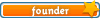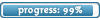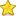Welcome

It is highly recommended that you have Javascript enabled; many features will not work unless you do. The recommended browsers are Firefox and Chrome; the board is also NOT mobile-friendly.

If you were referred by someone (ex. me, Jessica) please put their username in the referral box on the registration page. Ask them if you don't know their username.

If you are visiting for TESTING PURPOSES ONLY, this is the test account information:

Test

Select a forum to post in:

## Newton's Laws

Science topics

Moderators: Jessica, Teachers

Looking for a topic?
View the Science Topics Directory to find topics quicker under different kinds of science.

### Newton's Laws

Newton's First Law: The Law of Inertia
Provided there is no net force, a body at rest remains at rest, and a body in motion remains in motion with a constant velocity (constant speed AND direction). i.e. With no net force, there will be no acceleration.

Newton's Second Law: The Law of Acceleration
The acceleration of an object is directly proportional to the net force acting on it and inversely proportional to its mass. The direction of the acceleration is in the direction of the net force.

ΣF[sub]x[/sub] = ma[sub]x[/sub]
ΣF[sub]y[/sub] = ma[sub]y[/sub]

Force: cause
Acceleration: effect

Newton's Third Law: The Law of Interactions
Whenever one object exerts a force on a second object, the second exerts an equal and opposite force on the first.

The forces involved make up what is called an "action-reaction force pair". These forces act on two separate objects simultaneously.

ex. A force on a nail by a hammer.
A force on a hammer by a nail.

Free body diagrams show only forces acting ON an object. Thus the forces of the action-reaction force pair will NEVER be on the same diagram.

The EFFECT of a force can be different, even though the SIZE is the same.
• 0Jessica
Board Owner
Topic AuthorSapphirePosts: 3,470
Topics: 1,244
Articles: 30
Joined: December 22nd, 2010, 8:04 pm

### Re: Newton's Laws

Example (second law)

Two people are pushing a stalled car, as Figure 4.5a indicates. The mass of the car is 1850 kg. One person applies a force of 275 N to the car, while the other applies a force of 395 N. Both forces act in the same direction. A third force of 560 N also acts on the car, but in a direction opposite to that in which the people are pushing. This force arises because of friction and the extent to which the pavement opposes the motion of the tires. Find the acceleration of the car.

-image removed-

Reasoning
According to Newton's second law, the acceleration is the net force divided by the mass of the car. To determine the net force, we use the free-body diagram in Figure 4.5b. In this diagram, the car is represented as a dot, and its motion is along the x axis. The diagram makes it clear that the forces all act along one direction. Therefore, they can be added as colinear vectors to obtain the net force.

Solution
From Equation 4.1, the acceleration is -image removed-. The net force is
-image removed-
The acceleration can now be obtained: -image removed-

The plus sign indicates that the acceleration points along the x axis, in the same direction as the net force.
• 0Jessica
Board Owner
Topic AuthorSapphirePosts: 3,470
Topics: 1,244
Articles: 30
Joined: December 22nd, 2010, 8:04 pm